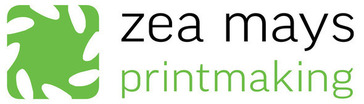/* styles */
 table div table+table+table div table{width:100%;padding:0}table div table+table+table div table img{width:96.23%;padding:0;float:none}table div table+table+table div table td{width:100%;padding:0 1.88% 18px}/* styles */# Printmaking in the time of Covid-19

 table div table+table+table+table+table+table div table{width:100%;padding:0}table div table+table+table+table+table+table div table img{width:96.23%;padding:0;float:none}table div table+table+table+table+table+table div table td{width:100%;padding:0 1.88% 18px}/* styles *//* styles */
 table div table+table+table+table+table+table+table+table div table{width:100%;padding:0}table div table+table+table+table+table+table+table+table div table img{width:96.23%;padding:0;float:none}table div table+table+table+table+table+table+table+table div table td{width:100%;padding:0 1.88% 18px}/* styles */## Liz Chalfin

How's everyone doing? I hope that you are healthy and staying safe.

First, I want to say a huge THANK YOU to any of you out there who are both readers of this newsletter, and people on the front lines of this pandemic - nurses, doctors, grocery store workers, postal workers, cleaners, bus drivers, newspaper publishers and delivery people, amazon workers, ups drivers....etc., etc. What would we do without you?! Several of our studio members are still working in these essential services and I think I can speak for everyone in our community when I give our deepest appreciation to you and your colleagues.

I also want to express my deepest gratitude to the members and friends of Zea Mays who have purchased gift certificates in the past couple of weeks. The outpouring of support and faith in the future of the studio has been incredible. It helps to cover the extensive losses we are now facing, and gives us hope that we will see many faces in the studio when we can physically reopen. There's still so much need. If you feel inclined, please purchase a gift certificate as a way of supporting the studio.

In the meantime, the studio faculty and I are working hard on developing some online courses -- video demos, virtual workshops, Zoom tutorials. We should have something to share very soon.

Another big thank you to our studio monitors Meredith Broberg, Angela Zammarelli and Pam Crawford who have been holding weekly Virtual Lunches with our members, near and far. It's been a fantastic way for people to check in with each other and share what they have been up to. It helps counter the isolation we are all feeling and keep us connected as a community.

We're uncertain when we can physically reopen our studio. When our Governor says it's safe, we'll reopen and hopefully people will feel confident to come back. We will be vigilant about cleaning and distancing as needed. Our presses are longing for some use!

The University of Massachusetts Arts Extension Services have put together a very comprehensive list of resources for artists and arts organizations. Thank you to them and so many others for gathering this information.

I've asked our members to share some of what they've been doing in isolation. Scroll down to the bottom of the email to get a glimpse of the creativity that continues, despite the challenges.

Much love,

Liz

 table div table+table+table+table+table+table+table+table+table+table+table div table{width:100%;padding:0}table div table+table+table+table+table+table+table+table+table+table+table div table img{width:96.23%;padding:0;float:none}table div table+table+table+table+table+table+table+table+table+table+table div table td{width:100%;padding:0 1.88% 18px}/* styles */## Online Videos and Workshops

Next week we'll begin rolling out a series of videos and virtual workshops presented by our fantastic faculty. You can look forward to:

▪ Making Inks from Natural Dyes with Marjorie Morgan
▪ Collage and Printmaking with Lynn Peterfreund
▪ Kitchen Table Monotype with Joyce Silverstone
▪ Nourishing Your Practice with Meredith Broberg
▪ Photo-based Vision Club with Nancy Diessner
▪ Woodcut with Julie Rivera
 ▪ Making Inks from Natural Dyes with Marjorie Morgan
 ▪ Collage and Printmaking with Lynn Peterfreund
 ▪ Kitchen Table Monotype with Joyce Silverstone
 ▪ Nourishing Your Practice with Meredith Broberg
 ▪ Photo-based Vision Club with Nancy Diessner
 ▪ Woodcut with Julie Rivera

with more to follow...

We will send out a separate mailing with details about how you can sign up for any of these offerings.

 table div table+table+table+table+table+table+table+table+table+table+table+table+table+table div table{width:100%;padding:0}table div table+table+table+table+table+table+table+table+table+table+table+table+table+table div table img{width:96.23%;padding:0;float:none}table div table+table+table+table+table+table+table+table+table+table+table+table+table+table div table td{width:100%;padding:0 1.88% 18px}/* styles */table div table+table+table+table+table+table+table+table+table+table+table+table+table+table+table+table div table,table.module-15{width:66.42%;float:right;padding:0}table div table+table+table+table+table+table+table+table+table+table+table+table+table+table+table+table div table a{border:0 none;text-decoration:none}table div table+table+table+table+table+table+table+table+table+table+table+table+table+table+table+table div table img{width:100%!important;border:0 none;text-decoration:none}table div table+table+table+table+table+table+table+table+table+table+table+table+table+table+table+table div table td{width:100%;padding:0 0 20px 20px}/* styles */ One way you can help Zea Mays Printmaking survive the pandemic is to purchase a gift certificate. They never expire and you can use them for a workshop, to buy some art from our Flat File Project, for a residency or a private lesson. Perhaps you know an essential worker who is also an artist and would love to get one as a thank you for the work they are doing. Or perhaps you just want to donate it back to the studio to use for scholarships. We'll gladly gift it to a printmaker in need.
 table div table+table+table+table+table+table+table+table+table+table+table+table+table+table+table+table+table+table div table{width:100%;padding:0}table div table+table+table+table+table+table+table+table+table+table+table+table+table+table+table+table+table+table div table img{width:96.23%;padding:0;float:none}table div table+table+table+table+table+table+table+table+table+table+table+table+table+table+table+table+table+table div table td{width:100%;padding:0 1.88% 18px}/* styles */## Print Day in May!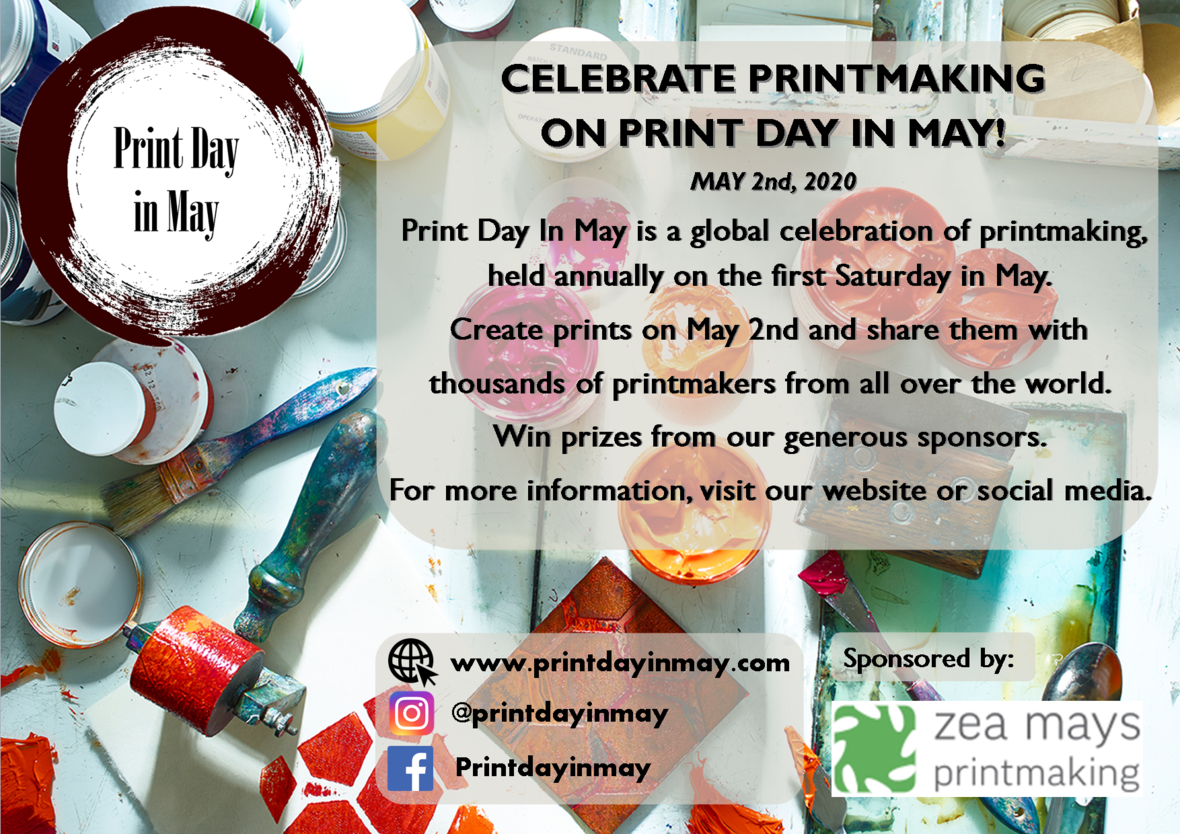Now More Than Ever.

Join tens of thousands of printmakers worldwide on May 2 for the annual 24 hour printmaking marathon that is Print Day in May. Let’s spread global joy and come together by doing what we love.

We are proud to be a sponsor of this year's Print Day in May. For details visit: Print Day in May.

 table div table+table+table+table+table+table+table+table+table+table+table+table+table+table+table+table+table+table+table+table+table div table{width:100%;padding:0}table div table+table+table+table+table+table+table+table+table+table+table+table+table+table+table+table+table+table+table+table+table div table img{width:96.23%;padding:0;float:none}table div table+table+table+table+table+table+table+table+table+table+table+table+table+table+table+table+table+table+table+table+table div table td{width:100%;padding:0 1.88% 18px}/* styles */## 2020 Residency Fellowship Winner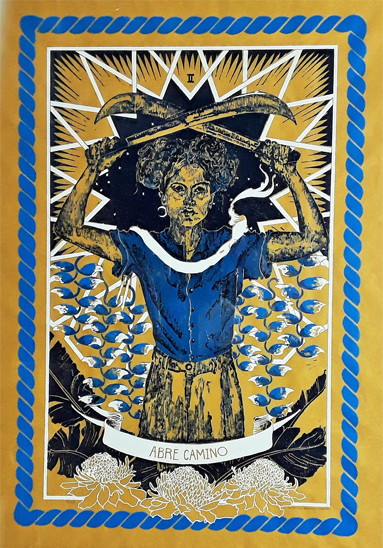We are thrilled to announce that Rosenda Alvarez Faro of Guaynabo, Puerto Rico is the winner of the 2020 Residency Fellowship.

Rosenda is co-director of Taller Malaquita and an active printmaker who works in linocut, woodcut and screen printing. Much of her work includes site specific wheat paste murals. When she comes to Zea Mays Printmaking we'll make our big brick building the site for one of her mural installations!

Of course everything is up in the air now, but we hope to have Rosenda at the studio in September for her residency.

 table div table+table+table+table+table+table+table+table+table+table+table+table+table+table+table+table+table+table+table+table+table+table+table+table div table{width:100%;padding:0}table div table+table+table+table+table+table+table+table+table+table+table+table+table+table+table+table+table+table+table+table+table+table+table+table div table img{width:96.23%;padding:0;float:none}table div table+table+table+table+table+table+table+table+table+table+table+table+table+table+table+table+table+table+table+table+table+table+table+table div table td{width:100%;padding:0 1.88% 18px}/* styles */# In Depth: Multiplicity of Meaning

Curated by Nancy Haver from the Zea Mays Printmaking Flat File Project 2020

Nancy writes: I love words that have multiple meanings, and the word depth seemed one well-suited to explore during this time of the pandemic–with its challenges of separation and of coping with one’s own grief, as well as grief for the rest of the world. This experience calls on us to reach far inside ourselves for resilience and examination–to plumb our depths.

See the exhibition here

 table div table+table+table+table+table+table+table+table+table+table+table+table+table+table+table+table+table+table+table+table+table+table+table+table+table+table+table div table{width:100%;padding:0}table div table+table+table+table+table+table+table+table+table+table+table+table+table+table+table+table+table+table+table+table+table+table+table+table+table+table+table div table img{width:96.23%;padding:0;float:none}table div table+table+table+table+table+table+table+table+table+table+table+table+table+table+table+table+table+table+table+table+table+table+table+table+table+table+table div table td{width:100%;padding:0 1.88% 18px}/* styles */## Exhibition Opportunities

 /* styles */ Print Club of Rochester - Juried International Call for Prints Exhibition Dates: June 5-July 25, 2020 Entry Deadline: April 19, 2020 Enter at: https://printclubofrochester.org/event/international-call-2020/ IPCNY New Prints Summer 2020 Entry Deadline: April 22, 2020 Enter at: https://ipcny.submittable.com/submit
 /* styles */ Edda Sigudardottir, Olwen Dowling, Elisa Lanzi, Scott McDaniel and Doris Madsen are included in the Hosmer Virtual Gallery. While Forbes Library is closed, Hosmer Gallery is inviting the community to participate in an online art exhibit. Everyone is welcome! Plus, you’re invited to contribute your original art to the gallery! Please send up to 3 images through the Virtual Hosmer Gallery Submission Form. Questions? email gallery@forbeslibrary.org.
 table div table+table+table+table+table+table+table+table+table+table+table+table+table+table+table+table+table+table+table+table+table+table+table+table+table+table+table+table+table+table+table div table{width:100%;padding:0}table div table+table+table+table+table+table+table+table+table+table+table+table+table+table+table+table+table+table+table+table+table+table+table+table+table+table+table+table+table+table+table div table img{width:96.23%;padding:0;float:none}table div table+table+table+table+table+table+table+table+table+table+table+table+table+table+table+table+table+table+table+table+table+table+table+table+table+table+table+table+table+table+table div table td{width:100%;padding:0 1.88% 18px}/* styles */# Snapshots from Covid-19 isolation studios

 table div table+table+table+table+table+table+table+table+table+table+table+table+table+table+table+table+table+table+table+table+table+table+table+table+table+table+table+table+table+table+table+table+table+table div table{width:100%;padding:0}table div table+table+table+table+table+table+table+table+table+table+table+table+table+table+table+table+table+table+table+table+table+table+table+table+table+table+table+table+table+table+table+table+table+table div table img{width:96.23%;padding:0;float:none}table div table+table+table+table+table+table+table+table+table+table+table+table+table+table+table+table+table+table+table+table+table+table+table+table+table+table+table+table+table+table+table+table+table+table div table td{width:100%;padding:0 1.88% 18px}/* styles *//* styles */ Elisa Lanzi is making art at Two Ponds Studio, her home in Belchertown, MA. With the onset of the pandemic, she began creating "collage stand-ups," petite portable diptychs that are meditations on connecting with solitude. Elisa is working on a new project with a local poet to create a publication featuring images paired with poems inspired by her art work. The poems will be in Spanish and English. Other current projects include prints for the 6x 6 2020 fundraising exhibition at Rochester Contemporary Art Center and conceptual models for the Zea Mays Green to the Extreme exhibition this fall. A spring show, Celestial: Art works by Sheryl Jaffe and Elisa Lanzi (Gibson Sotheby's International, Orleans, MA) was postponed and is being rescheduled. Elisa looks forward to pairing up with fellow handmade papermaker Sheryl Jaffe to teach the Pulp Dreams workshop at Zea Mays on June 6 and 7. Follow Elisa's posts on Instagram at @elisalanziartist and on her website www.elisalanzi.com.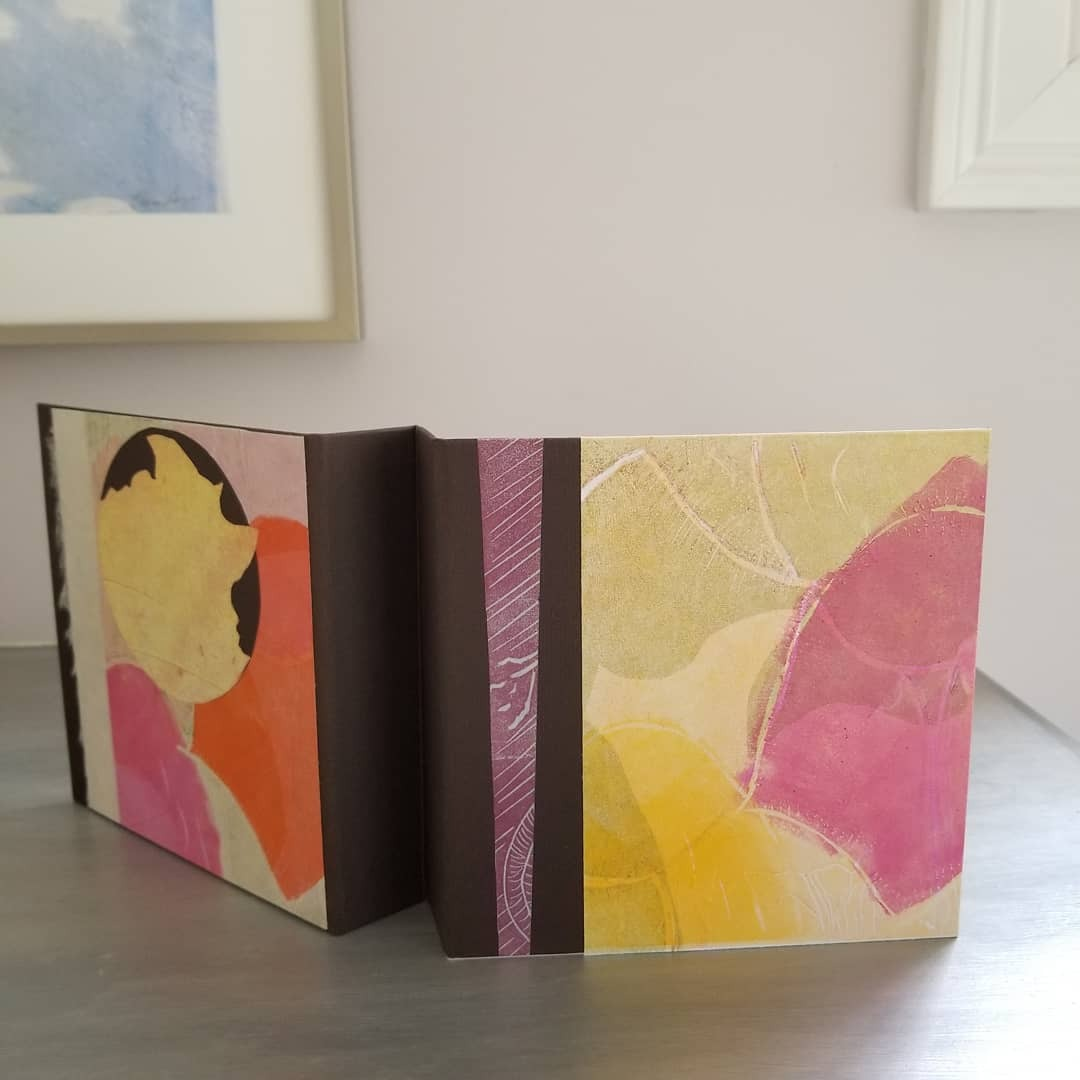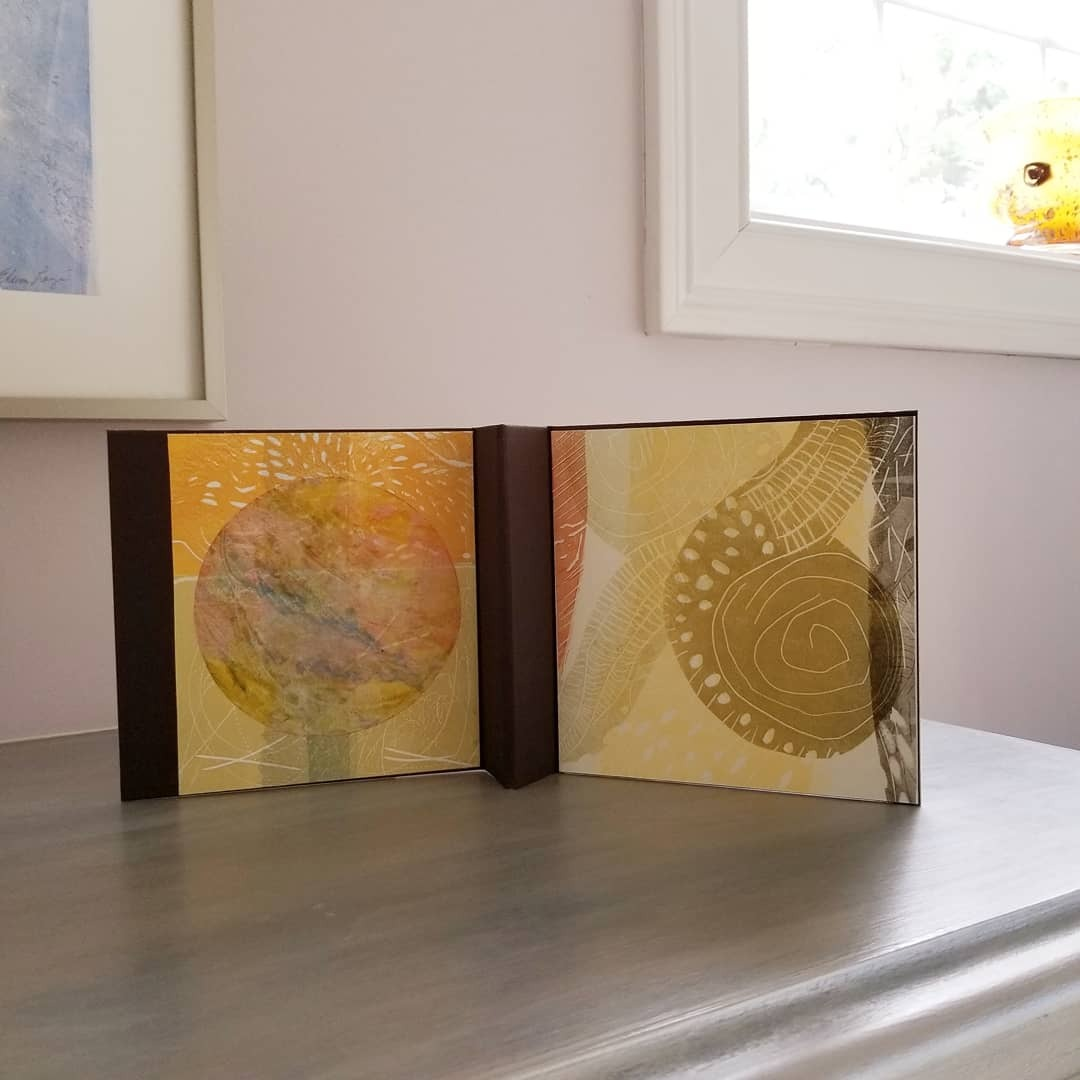table div table+table+table+table+table+table+table+table+table+table+table+table+table+table+table+table+table+table+table+table+table+table+table+table+table+table+table+table+table+table+table+table+table+table+table+table+table+table div table{width:100%;padding:0}table div table+table+table+table+table+table+table+table+table+table+table+table+table+table+table+table+table+table+table+table+table+table+table+table+table+table+table+table+table+table+table+table+table+table+table+table+table+table div table img{width:96.23%;padding:0;float:none}table div table+table+table+table+table+table+table+table+table+table+table+table+table+table+table+table+table+table+table+table+table+table+table+table+table+table+table+table+table+table+table+table+table+table+table+table+table+table div table td{width:100%;padding:0 1.88% 18px}/* styles *//* styles */ Julie Rivera Well at least we have more studio time! I’ve continued to work on my series from NYTimes’ Overlooked. Most recent additions are Sylvia Plath and Bessie Coleman (first African American female aviator).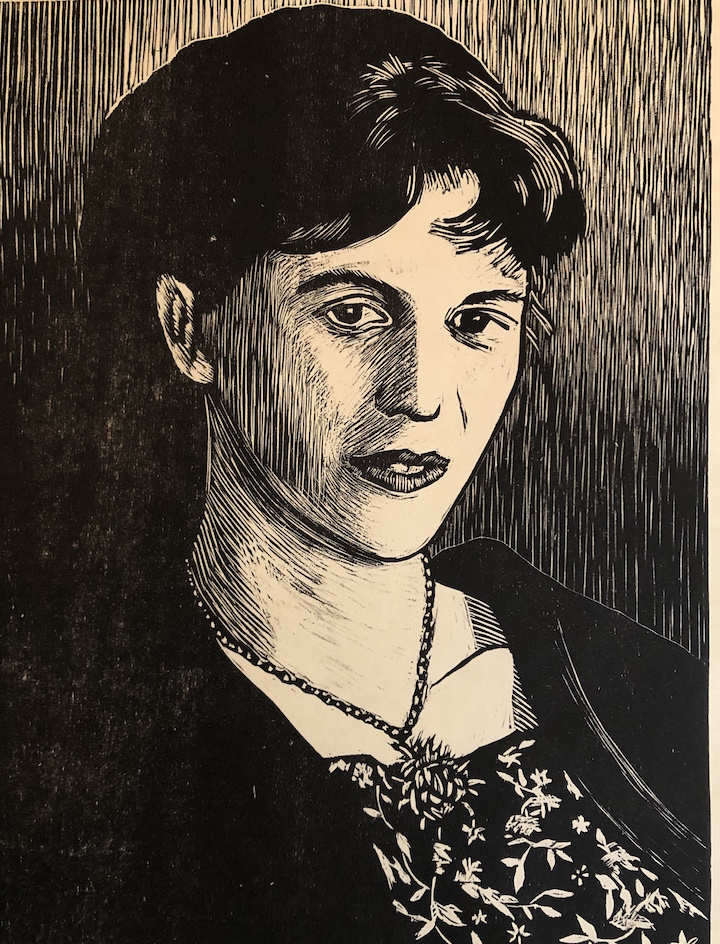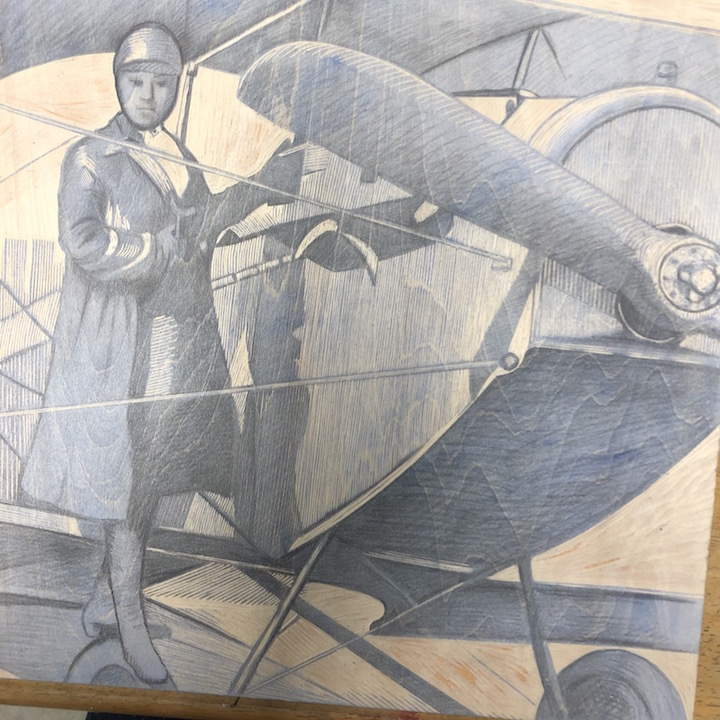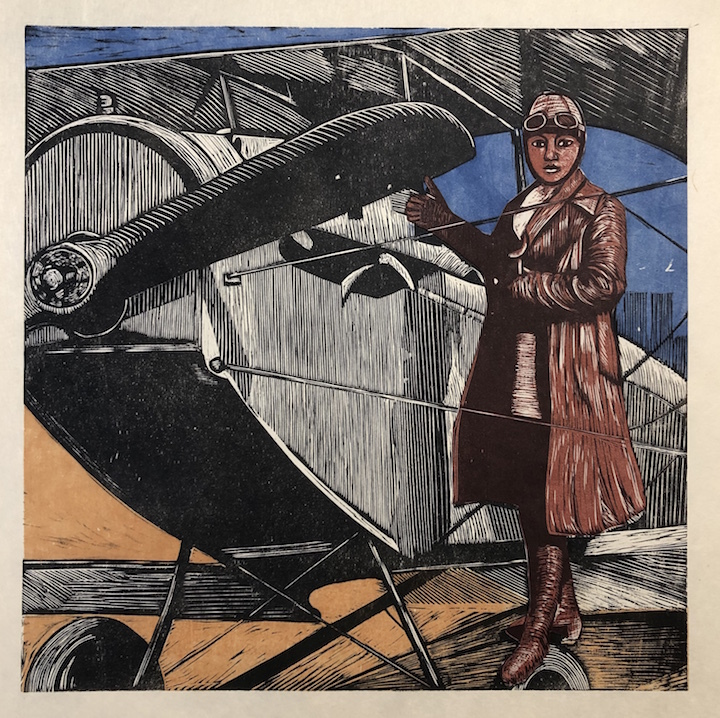table div table+table+table+table+table+table+table+table+table+table+table+table+table+table+table+table+table+table+table+table+table+table+table+table+table+table+table+table+table+table+table+table+table+table+table+table+table+table+table+table+table+table div table{width:100%;padding:0}table div table+table+table+table+table+table+table+table+table+table+table+table+table+table+table+table+table+table+table+table+table+table+table+table+table+table+table+table+table+table+table+table+table+table+table+table+table+table+table+table+table+table div table img{width:96.23%;padding:0;float:none}table div table+table+table+table+table+table+table+table+table+table+table+table+table+table+table+table+table+table+table+table+table+table+table+table+table+table+table+table+table+table+table+table+table+table+table+table+table+table+table+table+table+table div table td{width:100%;padding:0 1.88% 18px}/* styles *//* styles */ Angela Earley My studio practice helps me to maintain a universal perspective while living in isolation.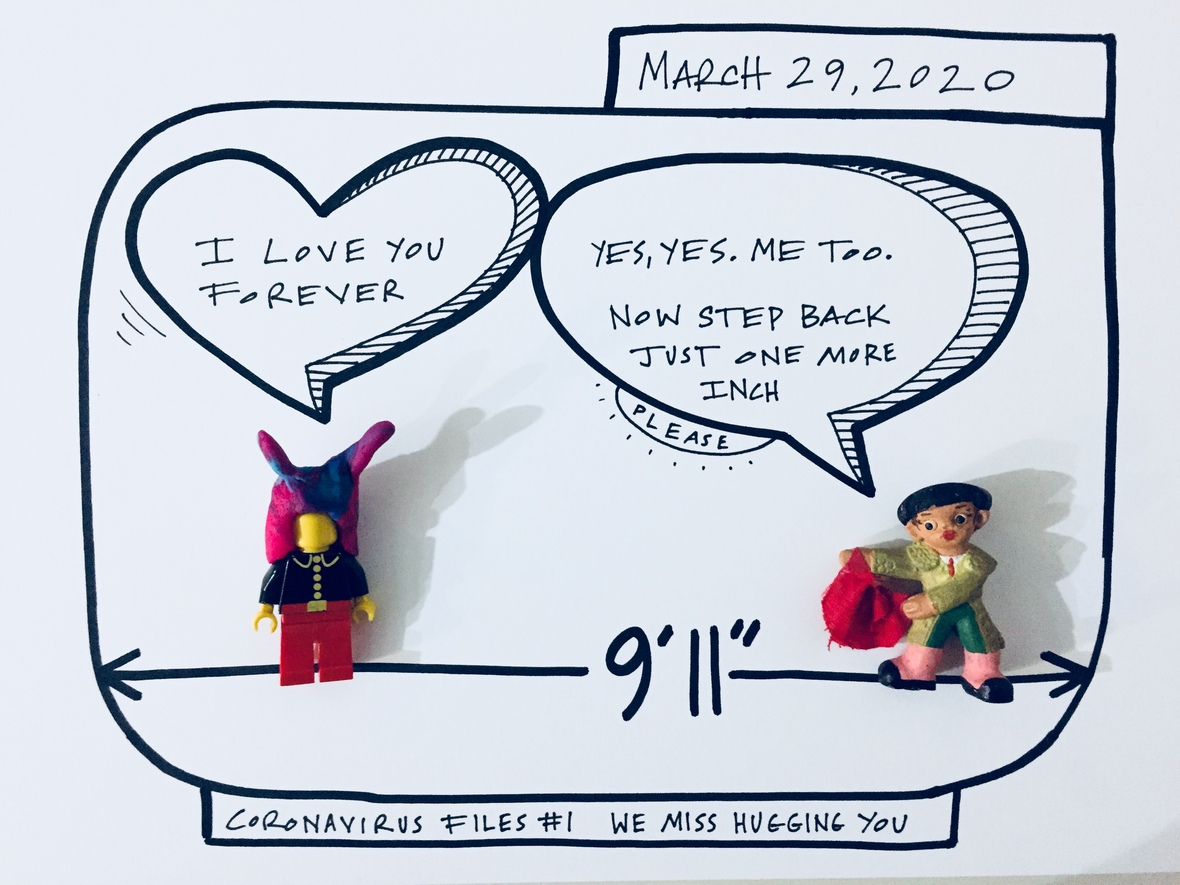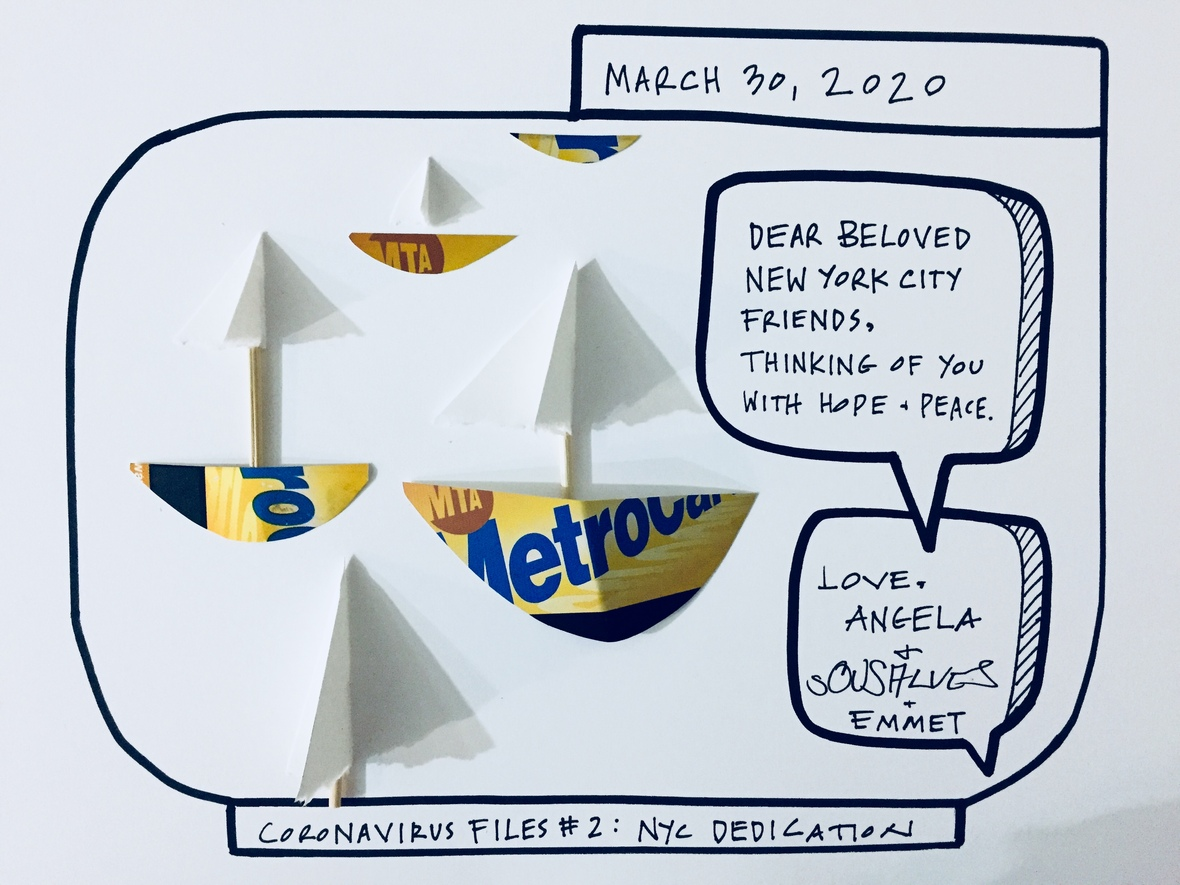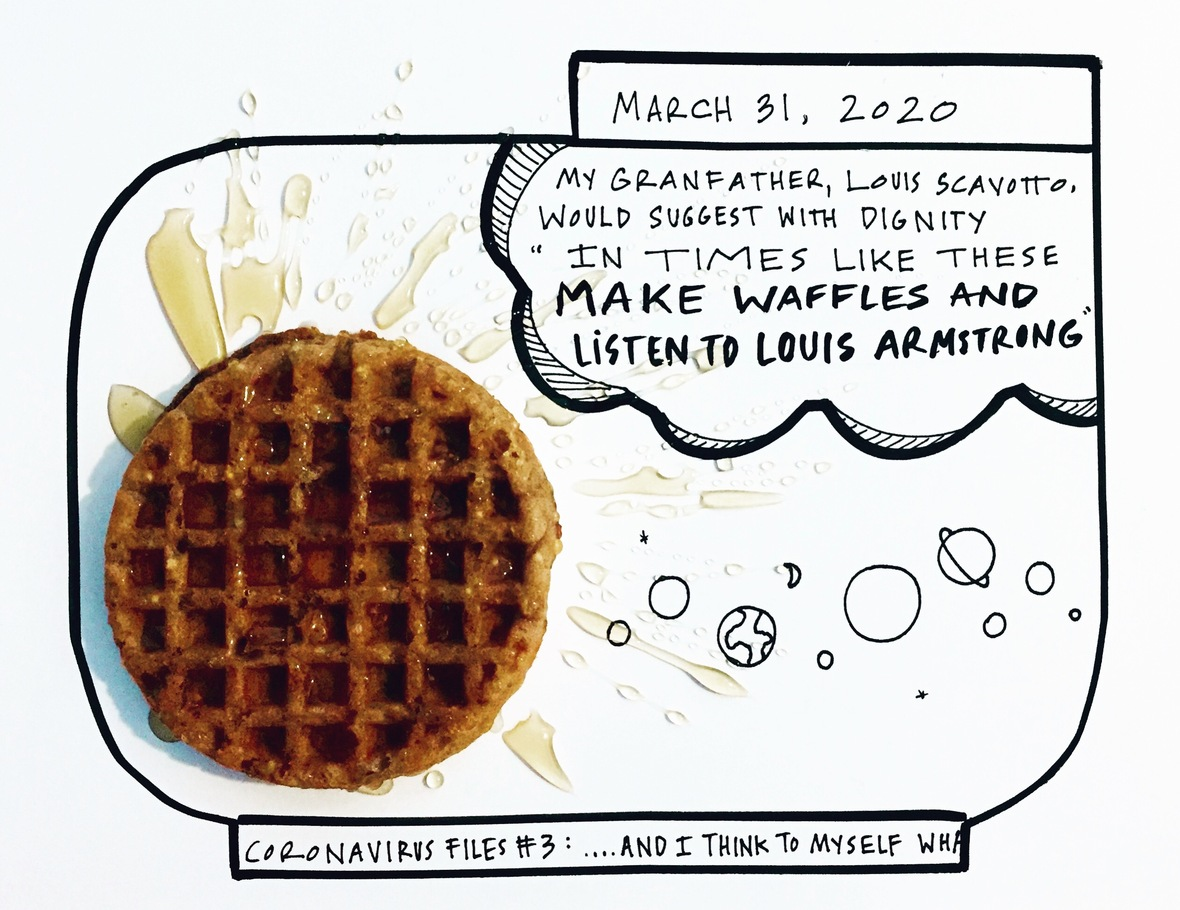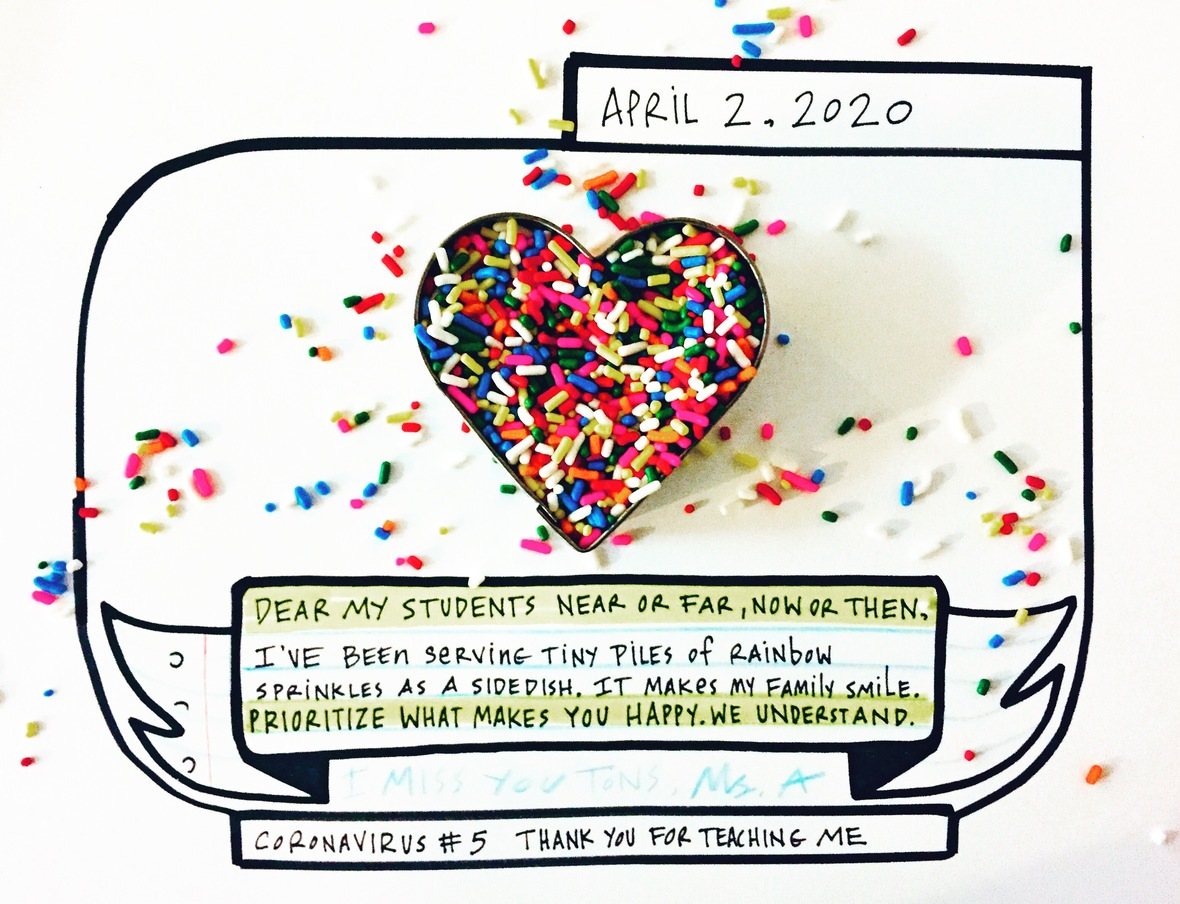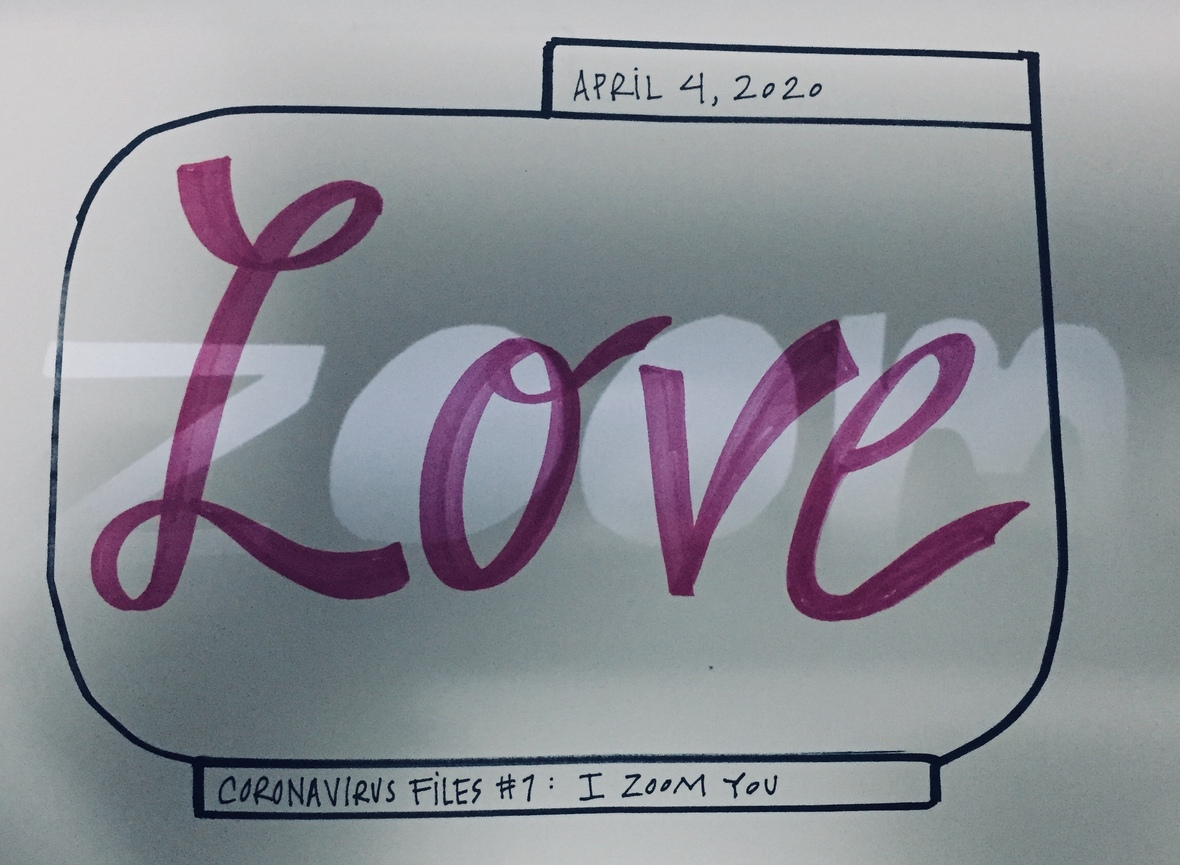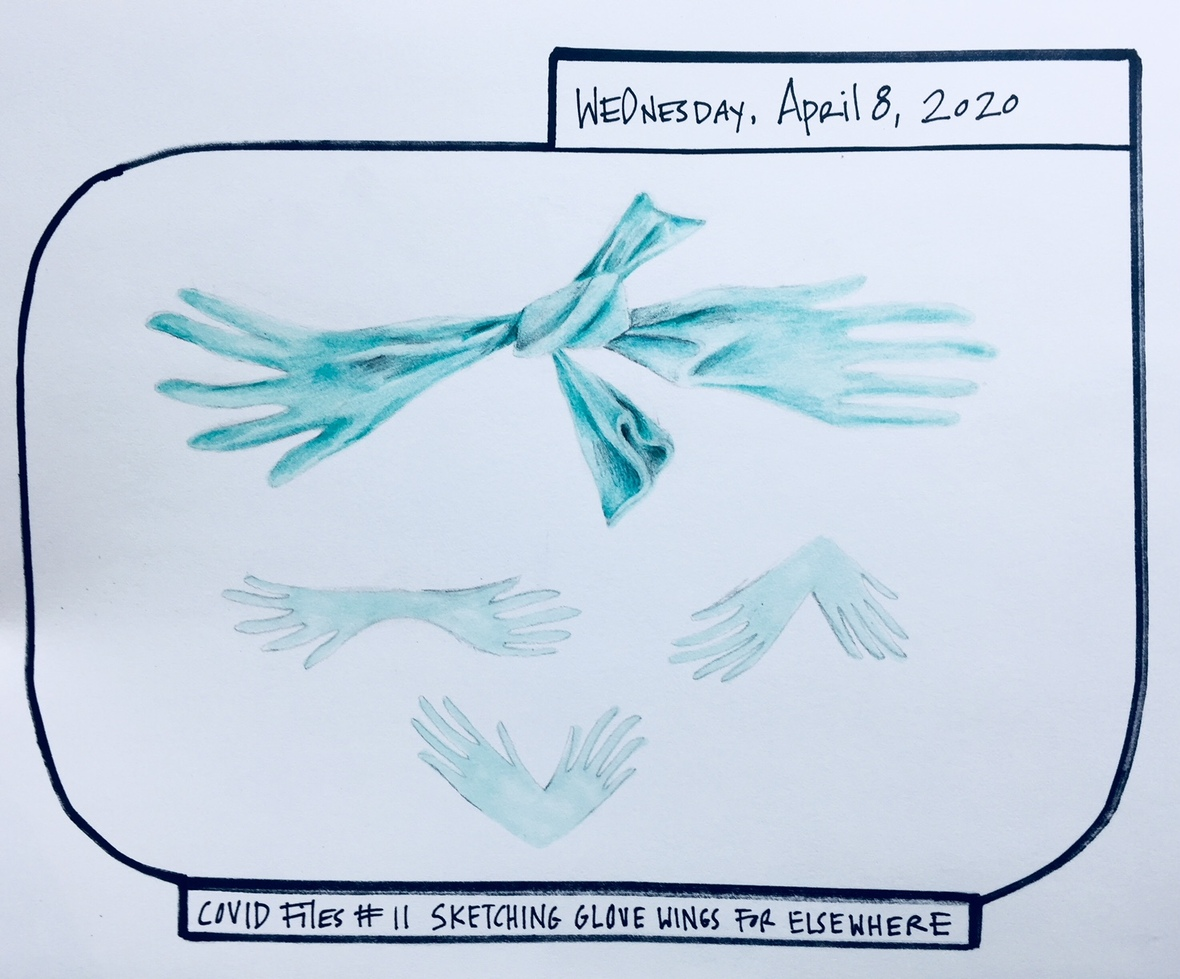table div table+table+table+table+table+table+table+table+table+table+table+table+table+table+table+table+table+table+table+table+table+table+table+table+table+table+table+table+table+table+table+table+table+table+table+table+table+table+table+table+table+table+table+table+table+table+table+table+table+table div table{width:100%;padding:0}table div table+table+table+table+table+table+table+table+table+table+table+table+table+table+table+table+table+table+table+table+table+table+table+table+table+table+table+table+table+table+table+table+table+table+table+table+table+table+table+table+table+table+table+table+table+table+table+table+table+table div table img{width:96.23%;padding:0;float:none}table div table+table+table+table+table+table+table+table+table+table+table+table+table+table+table+table+table+table+table+table+table+table+table+table+table+table+table+table+table+table+table+table+table+table+table+table+table+table+table+table+table+table+table+table+table+table+table+table+table+table div table td{width:100%;padding:0 1.88% 18px}/* styles *//* styles */ Peggy Merritt The "Canary in a Coal Mine" project has shaped most of my recent work in preparation for using the 4.5" x 6" copper plate that you have sent me. (I have a stash of 4" x 6" plates for some of studies.) Not only has the project encouraged me to return to copper plates and traditional techniques--etching, drypoint, chine colle, and aquatint--but also has helped me learn about the highly conserved arrangement of feathers on all birds, from turkeys to warblers. I have focused on three species whose range is threatened in my part of NH: evening grosbeak, black-throated blue warbler, and scarlet tanager.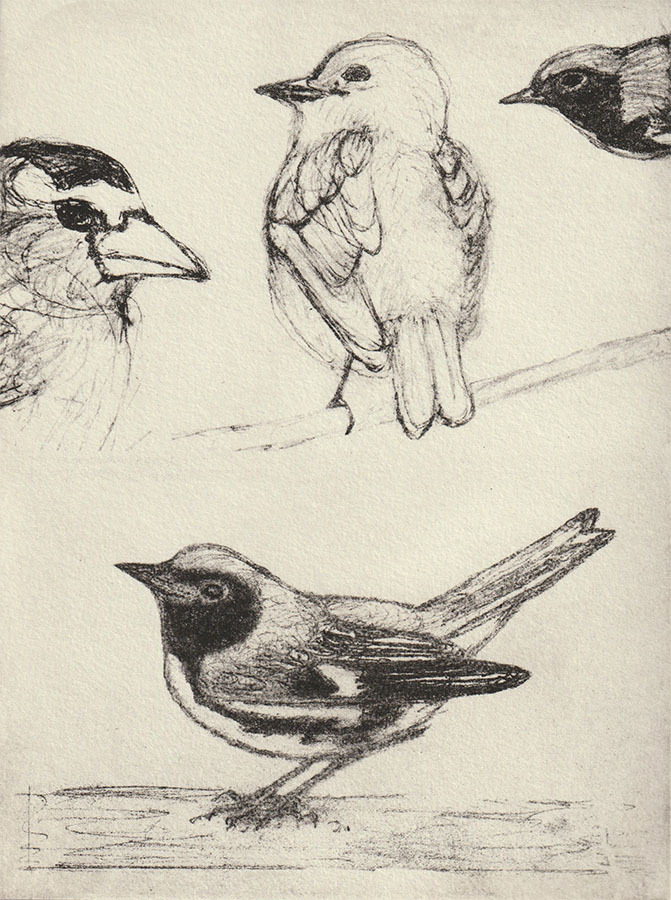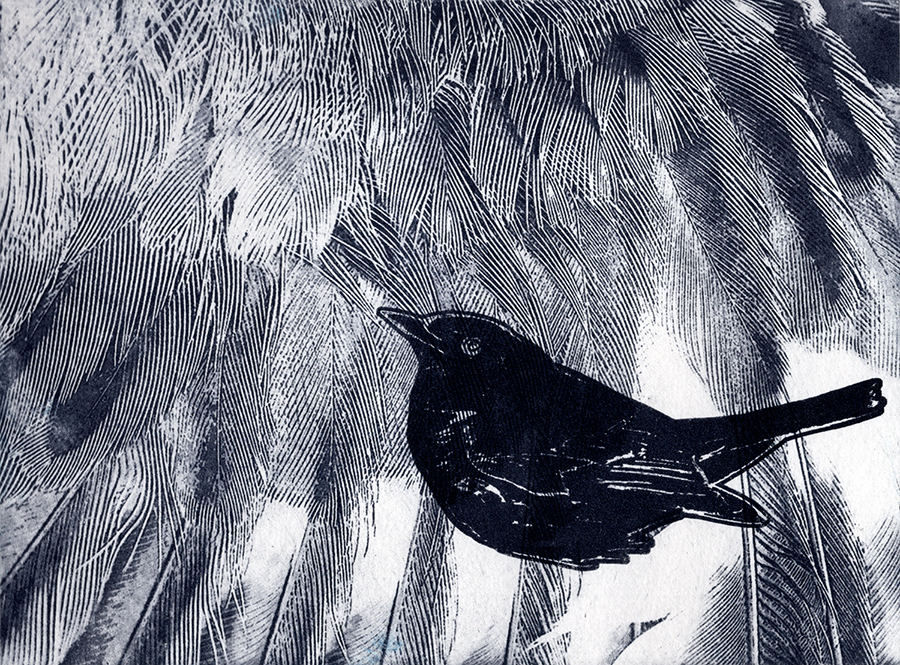table div table+table+table+table+table+table+table+table+table+table+table+table+table+table+table+table+table+table+table+table+table+table+table+table+table+table+table+table+table+table+table+table+table+table+table+table+table+table+table+table+table+table+table+table+table+table+table+table+table+table+table+table+table+table div table{width:100%;padding:0}table div table+table+table+table+table+table+table+table+table+table+table+table+table+table+table+table+table+table+table+table+table+table+table+table+table+table+table+table+table+table+table+table+table+table+table+table+table+table+table+table+table+table+table+table+table+table+table+table+table+table+table+table+table+table div table img{width:96.23%;padding:0;float:none}table div table+table+table+table+table+table+table+table+table+table+table+table+table+table+table+table+table+table+table+table+table+table+table+table+table+table+table+table+table+table+table+table+table+table+table+table+table+table+table+table+table+table+table+table+table+table+table+table+table+table+table+table+table+table div table td{width:100%;padding:0 1.88% 18px}/* styles *//* styles */ Lyell Castonguay is currently working on this 2 by 4 foot woodblock. It is from a series that pays tribute to the natural world and the ways humans continue to shape the environment.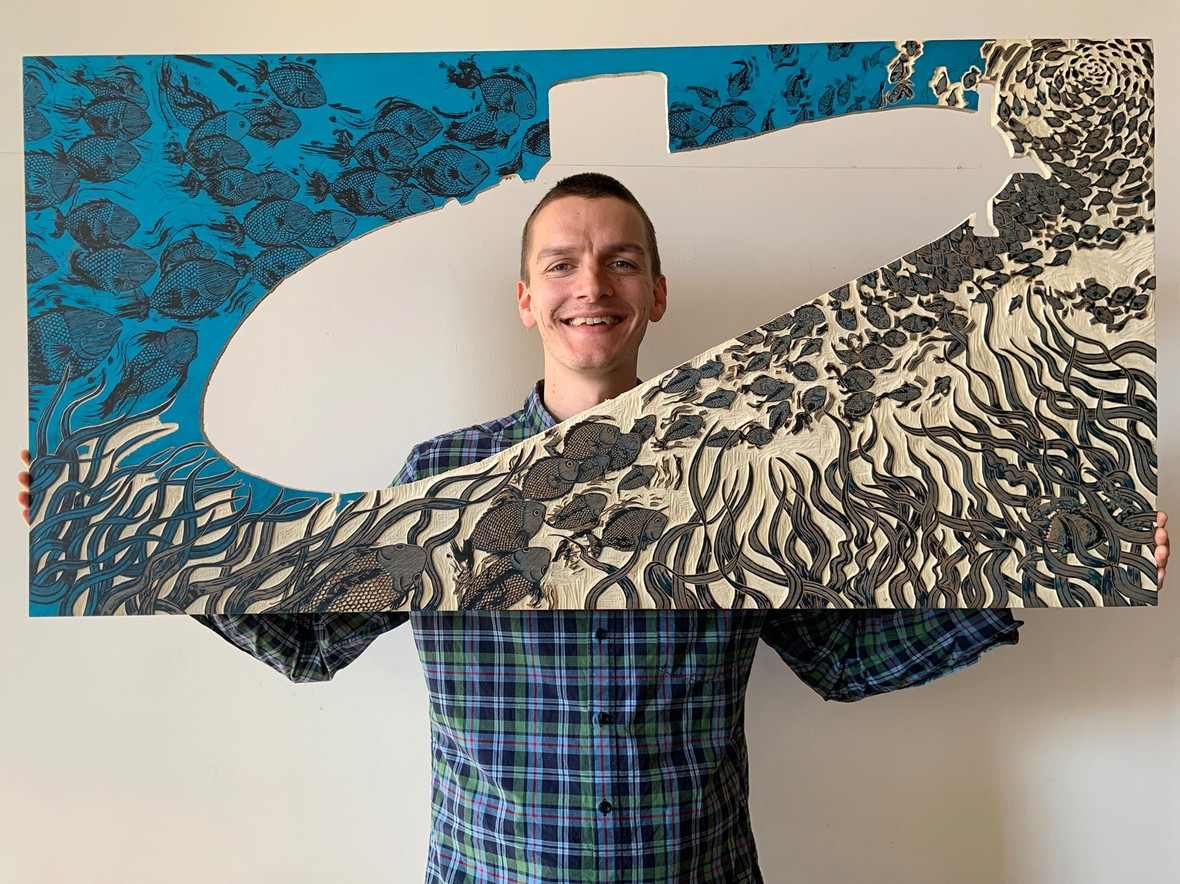table div table+table+table+table+table+table+table+table+table+table+table+table+table+table+table+table+table+table+table+table+table+table+table+table+table+table+table+table+table+table+table+table+table+table+table+table+table+table+table+table+table+table+table+table+table+table+table+table+table+table+table+table+table+table+table+table+table div table{width:100%;padding:0}table div table+table+table+table+table+table+table+table+table+table+table+table+table+table+table+table+table+table+table+table+table+table+table+table+table+table+table+table+table+table+table+table+table+table+table+table+table+table+table+table+table+table+table+table+table+table+table+table+table+table+table+table+table+table+table+table+table div table img{width:96.23%;padding:0;float:none}table div table+table+table+table+table+table+table+table+table+table+table+table+table+table+table+table+table+table+table+table+table+table+table+table+table+table+table+table+table+table+table+table+table+table+table+table+table+table+table+table+table+table+table+table+table+table+table+table+table+table+table+table+table+table+table+table+table div table td{width:100%;padding:0 1.88% 18px}/* styles *//* styles */ Annie Bissett (Providence RI) has been working on a woodblock print tarot deck for over a year, and that project continues since she works mainly in a home-based studio. Pictured are the three “Major Arcana” cards that she has completed over the past four weeks during Rhode Island’s stay-at-home order. You can follow Woodblock Dreams Tarot on Instagram to see the previous 56 cards and follow Annie’s progress as she works to complete all 78 images. The deck will eventually be commercially published for sale.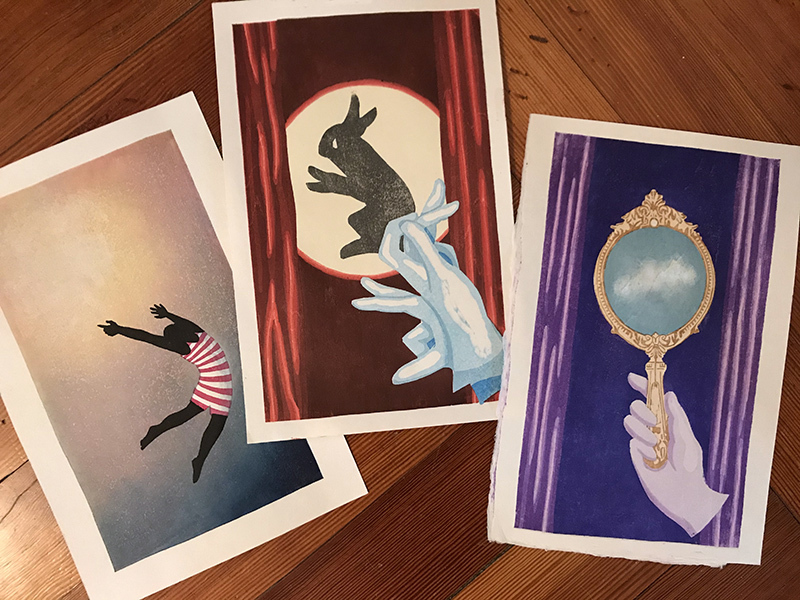table div table+table+table+table+table+table+table+table+table+table+table+table+table+table+table+table+table+table+table+table+table+table+table+table+table+table+table+table+table+table+table+table+table+table+table+table+table+table+table+table+table+table+table+table+table+table+table+table+table+table+table+table+table+table+table+table+table+table+table+table div table{width:100%;padding:0}table div table+table+table+table+table+table+table+table+table+table+table+table+table+table+table+table+table+table+table+table+table+table+table+table+table+table+table+table+table+table+table+table+table+table+table+table+table+table+table+table+table+table+table+table+table+table+table+table+table+table+table+table+table+table+table+table+table+table+table+table div table img{width:96.23%;padding:0;float:none}table div table+table+table+table+table+table+table+table+table+table+table+table+table+table+table+table+table+table+table+table+table+table+table+table+table+table+table+table+table+table+table+table+table+table+table+table+table+table+table+table+table+table+table+table+table+table+table+table+table+table+table+table+table+table+table+table+table+table+table+table div table td{width:100%;padding:0 1.88% 18px}/* styles *//* styles */ Marjorie Morgan has been expanding her knowledge of ink-making by creating lots of new inks and expanding into the field of Lake Pigment (ink that have been turned into pigment). She misses the ZMP community and having access to a press, but she is playing around with hand printing in her home studio and painting over previously-made prints. Marjorie is also working on a series of video tutorials for Zea Mays about creating inks and using them in a monotype printmaking process. You can see her regular posts on Instagram by following @marjart or explore her website, www.marjoriemorgan.net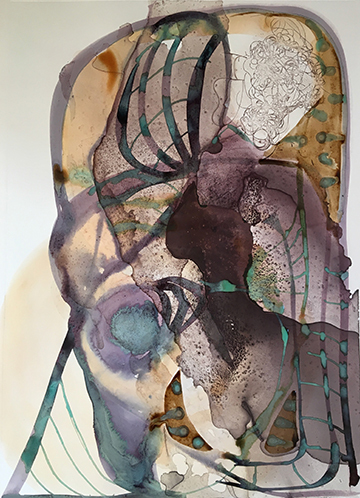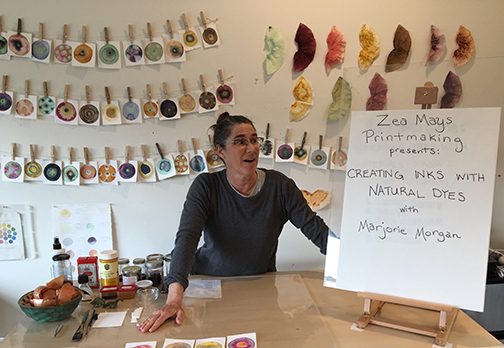table div table+table+table+table+table+table+table+table+table+table+table+table+table+table+table+table+table+table+table+table+table+table+table+table+table+table+table+table+table+table+table+table+table+table+table+table+table+table+table+table+table+table+table+table+table+table+table+table+table+table+table+table+table+table+table+table+table+table+table+table+table+table+table+table div table{width:100%;padding:0}table div table+table+table+table+table+table+table+table+table+table+table+table+table+table+table+table+table+table+table+table+table+table+table+table+table+table+table+table+table+table+table+table+table+table+table+table+table+table+table+table+table+table+table+table+table+table+table+table+table+table+table+table+table+table+table+table+table+table+table+table+table+table+table+table div table img{width:96.23%;padding:0;float:none}table div table+table+table+table+table+table+table+table+table+table+table+table+table+table+table+table+table+table+table+table+table+table+table+table+table+table+table+table+table+table+table+table+table+table+table+table+table+table+table+table+table+table+table+table+table+table+table+table+table+table+table+table+table+table+table+table+table+table+table+table+table+table+table+table div table td{width:100%;padding:0 1.88% 18px}/* styles *//* styles */ Martha Braun Image Drawn From Word Extracted From the Goldmine of My Heart Knocking on the Door of the Abyss Black Cats and Spiders Have My Back Reading Blank Pages in the Dark That Which Once Was Will Come Again Before the Present There Was the Past and the Future My Heart, My Core, My Strength : The Apple Tree I often start with words to get to imagery. These seven phrases may be titles but are certainly pathways into the imagery I am looking to create.....carve, print. Or perhaps the words themselves will become the image. Doing the work will determine the outcome.
 table div table+table+table+table+table+table+table+table+table+table+table+table+table+table+table+table+table+table+table+table+table+table+table+table+table+table+table+table+table+table+table+table+table+table+table+table+table+table+table+table+table+table+table+table+table+table+table+table+table+table+table+table+table+table+table+table+table+table+table+table+table+table+table+table+table+table div table{width:100%;padding:0}table div table+table+table+table+table+table+table+table+table+table+table+table+table+table+table+table+table+table+table+table+table+table+table+table+table+table+table+table+table+table+table+table+table+table+table+table+table+table+table+table+table+table+table+table+table+table+table+table+table+table+table+table+table+table+table+table+table+table+table+table+table+table+table+table+table+table div table img{width:96.23%;padding:0;float:none}table div table+table+table+table+table+table+table+table+table+table+table+table+table+table+table+table+table+table+table+table+table+table+table+table+table+table+table+table+table+table+table+table+table+table+table+table+table+table+table+table+table+table+table+table+table+table+table+table+table+table+table+table+table+table+table+table+table+table+table+table+table+table+table+table+table+table div table td{width:100%;padding:0 1.88% 18px}/* styles *//* styles */ Liz Chalfin and Sheldon Carroll returned from a residency at the Scuola Internazionale de Grafica in Venice, Italy on March 7th. In Italy, we began a print project that used the amazing architectural sculptures around the city as inspiration, predominantly the mascaroni, or keystones that hold up the arches. These are photo etchings with chine colle. We are working on finishing up the series here at home and making an artists' book as well as individual prints.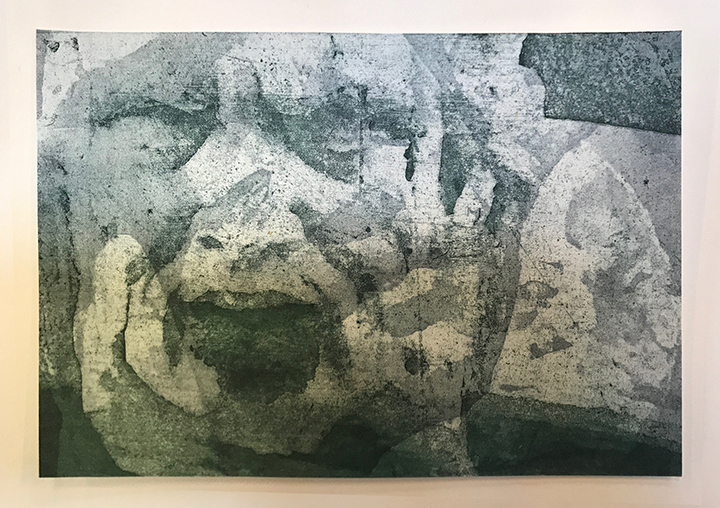table div table+table+table+table+table+table+table+table+table+table+table+table+table+table+table+table+table+table+table+table+table+table+table+table+table+table+table+table+table+table+table+table+table+table+table+table+table+table+table+table+table+table+table+table+table+table+table+table+table+table+table+table+table+table+table+table+table+table+table+table+table+table+table+table+table+table+table+table+table div table{width:100%;padding:0}table div table+table+table+table+table+table+table+table+table+table+table+table+table+table+table+table+table+table+table+table+table+table+table+table+table+table+table+table+table+table+table+table+table+table+table+table+table+table+table+table+table+table+table+table+table+table+table+table+table+table+table+table+table+table+table+table+table+table+table+table+table+table+table+table+table+table+table+table+table div table img{width:96.23%;padding:0;float:none}table div table+table+table+table+table+table+table+table+table+table+table+table+table+table+table+table+table+table+table+table+table+table+table+table+table+table+table+table+table+table+table+table+table+table+table+table+table+table+table+table+table+table+table+table+table+table+table+table+table+table+table+table+table+table+table+table+table+table+table+table+table+table+table+table+table+table+table+table+table div table td{width:100%;padding:0 1.88% 18px}/* styles *//* styles */ Anita Hunt can’t stream or zoom, video chat or text from her rural quarantine location but she is posting daily observations and drawings in progress on Instagram @batworthy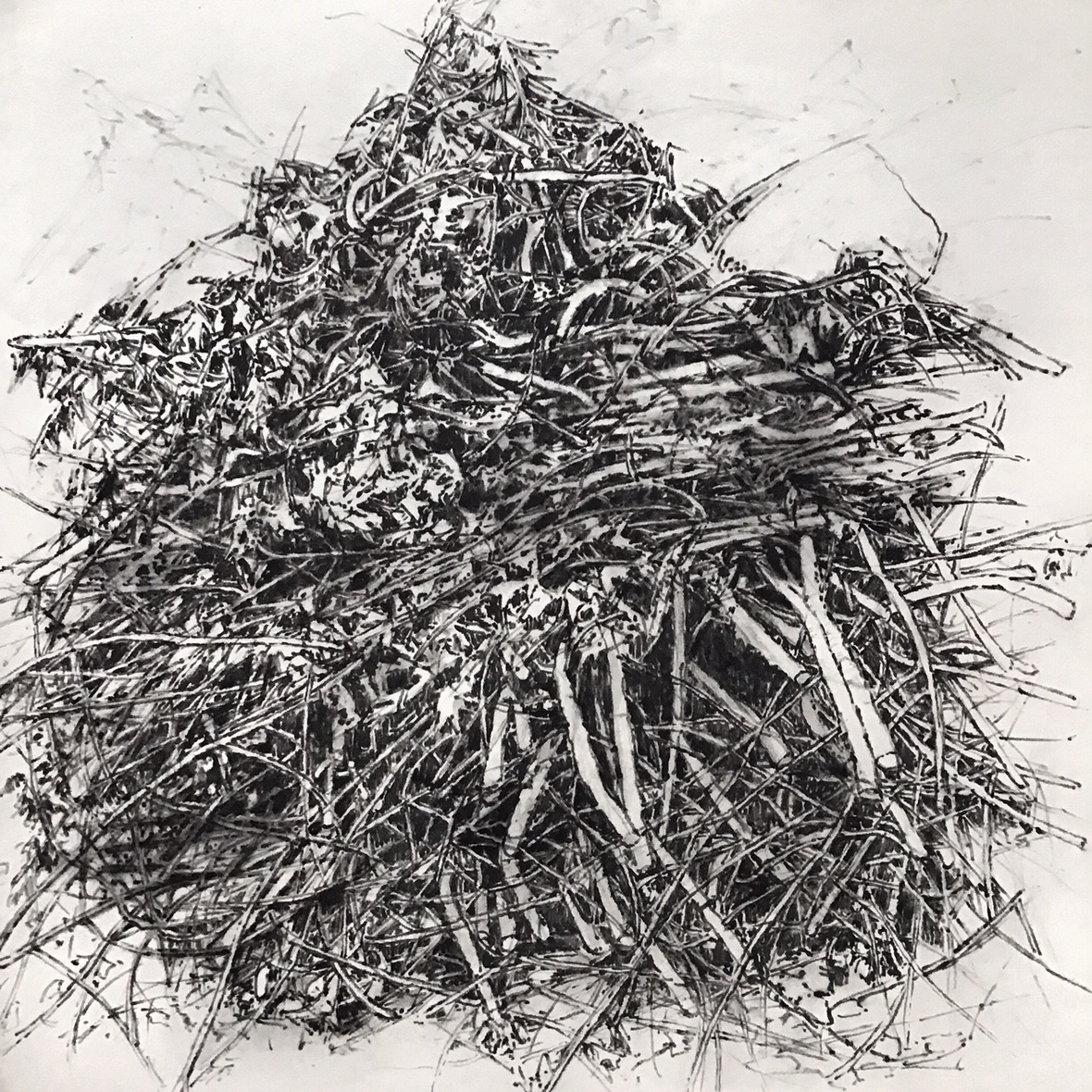table div table+table+table+table+table+table+table+table+table+table+table+table+table+table+table+table+table+table+table+table+table+table+table+table+table+table+table+table+table+table+table+table+table+table+table+table+table+table+table+table+table+table+table+table+table+table+table+table+table+table+table+table+table+table+table+table+table+table+table+table+table+table+table+table+table+table+table+table+table+table+table+table div table{width:100%;padding:0}table div table+table+table+table+table+table+table+table+table+table+table+table+table+table+table+table+table+table+table+table+table+table+table+table+table+table+table+table+table+table+table+table+table+table+table+table+table+table+table+table+table+table+table+table+table+table+table+table+table+table+table+table+table+table+table+table+table+table+table+table+table+table+table+table+table+table+table+table+table+table+table+table div table img{width:96.23%;padding:0;float:none}table div table+table+table+table+table+table+table+table+table+table+table+table+table+table+table+table+table+table+table+table+table+table+table+table+table+table+table+table+table+table+table+table+table+table+table+table+table+table+table+table+table+table+table+table+table+table+table+table+table+table+table+table+table+table+table+table+table+table+table+table+table+table+table+table+table+table+table+table+table+table+table+table div table td{width:100%;padding:0 1.88% 18px}/* styles */NC Whitcher, CT Monotype Printmaker

1. Juror, Dr. Courtney J. Martin selected Monoprint “and another” for the 116th Annual New Haven Paint and Clay Club Exhibition..online exhibition: May 3-May 31 the Club's website at: https://www.newhavenpaintandclayclub.org/ and on DailyBrushwork.Com at http://dailybrushwork.com/Default.aspx
2. Juror, Jean Dalton selected “Summer" and “Red” for the Connecticut Women Artist’s: CWA Member’s Juried Show…online show: www.ctwomenartists.org and on dailybrushwork.com “an another".
3. “Red” and “Landscape" showing until April 28th at #Local Gallery in Easthampton, MAss for the MGNE Invitational Exhibit: Singular” Monotypes and Monoprints
 1 Juror, Dr. Courtney J. Martin selected Monoprint “and another” for the 116th Annual New Haven Paint and Clay Club Exhibition..online exhibition: May 3-May 31 the Club's website at: https://www.newhavenpaintandclayclub.org/ and on DailyBrushwork.Com at http://dailybrushwork.com/Default.aspx
 2 Juror, Jean Dalton selected “Summer" and “Red” for the Connecticut Women Artist’s: CWA Member’s Juried Show…online show: www.ctwomenartists.org and on dailybrushwork.com “an another".
 3 “Red” and “Landscape" showing until April 28th at #Local Gallery in Easthampton, MAss for the MGNE Invitational Exhibit: Singular” Monotypes and Monoprints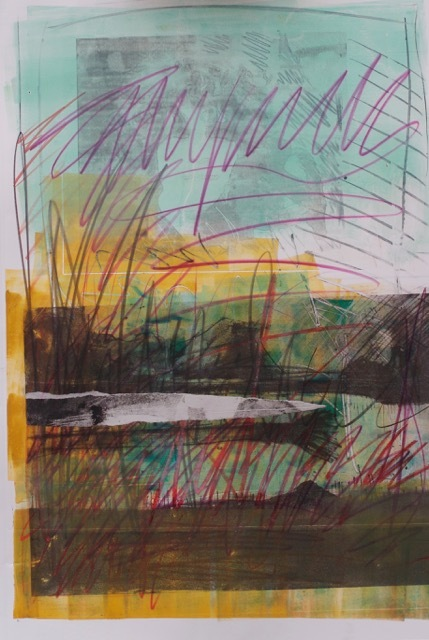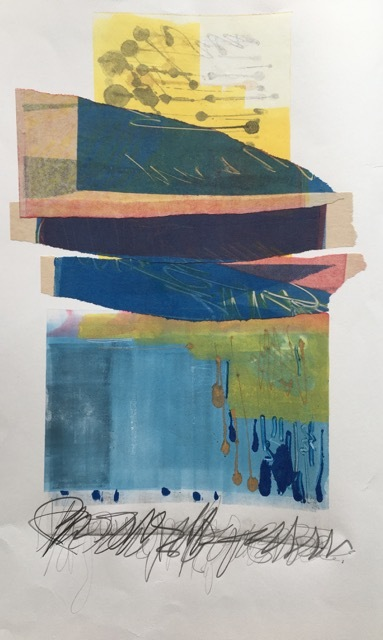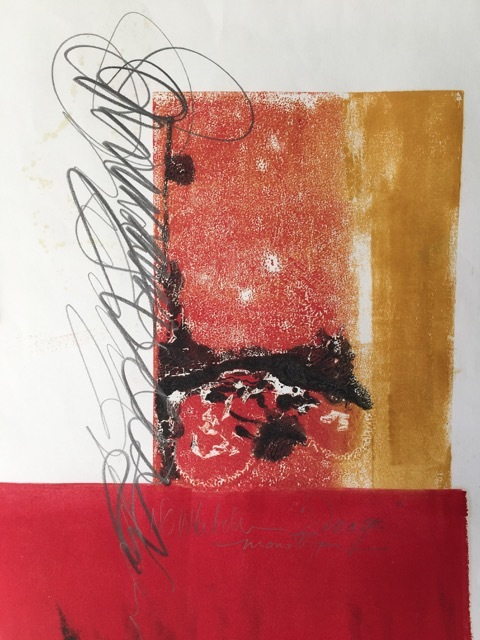table div table+table+table+table+table+table+table+table+table+table+table+table+table+table+table+table+table+table+table+table+table+table+table+table+table+table+table+table+table+table+table+table+table+table+table+table+table+table+table+table+table+table+table+table+table+table+table+table+table+table+table+table+table+table+table+table+table+table+table+table+table+table+table+table+table+table+table+table+table+table+table+table+table+table+table+table+table div table{width:100%;padding:0}table div table+table+table+table+table+table+table+table+table+table+table+table+table+table+table+table+table+table+table+table+table+table+table+table+table+table+table+table+table+table+table+table+table+table+table+table+table+table+table+table+table+table+table+table+table+table+table+table+table+table+table+table+table+table+table+table+table+table+table+table+table+table+table+table+table+table+table+table+table+table+table+table+table+table+table+table+table div table img{width:96.23%;padding:0;float:none}table div table+table+table+table+table+table+table+table+table+table+table+table+table+table+table+table+table+table+table+table+table+table+table+table+table+table+table+table+table+table+table+table+table+table+table+table+table+table+table+table+table+table+table+table+table+table+table+table+table+table+table+table+table+table+table+table+table+table+table+table+table+table+table+table+table+table+table+table+table+table+table+table+table+table+table+table+table div table td{width:100%;padding:0 1.88% 18px}/* styles *//* styles */ Susan Rood, "I'm using a grid structure when combining my layered square linocuts to create larger works. This more formal approach to composition is helping me find some order during a very strange and chaotic time." https://www.instagram.com/susanroodarts/ http://www.facebook.com/pages/Susan-Rood-Art/156094444420648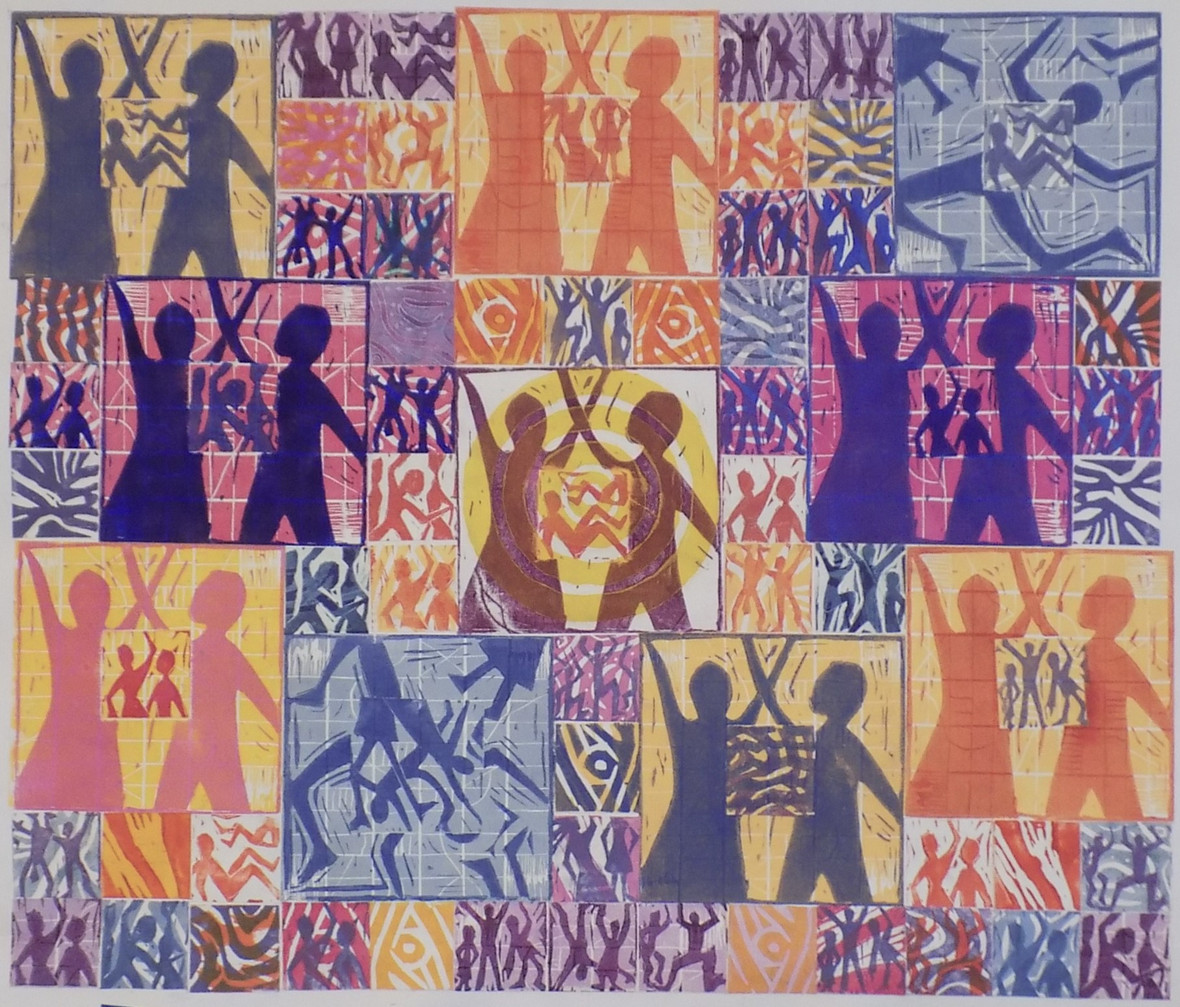table div table+table+table+table+table+table+table+table+table+table+table+table+table+table+table+table+table+table+table+table+table+table+table+table+table+table+table+table+table+table+table+table+table+table+table+table+table+table+table+table+table+table+table+table+table+table+table+table+table+table+table+table+table+table+table+table+table+table+table+table+table+table+table+table+table+table+table+table+table+table+table+table+table+table+table+table+table+table+table+table div table{width:100%;padding:0}table div table+table+table+table+table+table+table+table+table+table+table+table+table+table+table+table+table+table+table+table+table+table+table+table+table+table+table+table+table+table+table+table+table+table+table+table+table+table+table+table+table+table+table+table+table+table+table+table+table+table+table+table+table+table+table+table+table+table+table+table+table+table+table+table+table+table+table+table+table+table+table+table+table+table+table+table+table+table+table+table div table img{width:96.23%;padding:0;float:none}table div table+table+table+table+table+table+table+table+table+table+table+table+table+table+table+table+table+table+table+table+table+table+table+table+table+table+table+table+table+table+table+table+table+table+table+table+table+table+table+table+table+table+table+table+table+table+table+table+table+table+table+table+table+table+table+table+table+table+table+table+table+table+table+table+table+table+table+table+table+table+table+table+table+table+table+table+table+table+table+table div table td{width:100%;padding:0 1.88% 18px}/* styles *//* styles */ Tina Stevens Here is project I am doing using monoprinted design elements that I scan into digital format. In Photoshop I do a layout and create different colorways. I like to make a paper comp to see how things look to scale. This project is for pillows inspired by the beach roses and hips I see at the Cape. I send the files out for digital printing, this is cotton/linen and just arrived to me today. Next steps are cutting and sewing.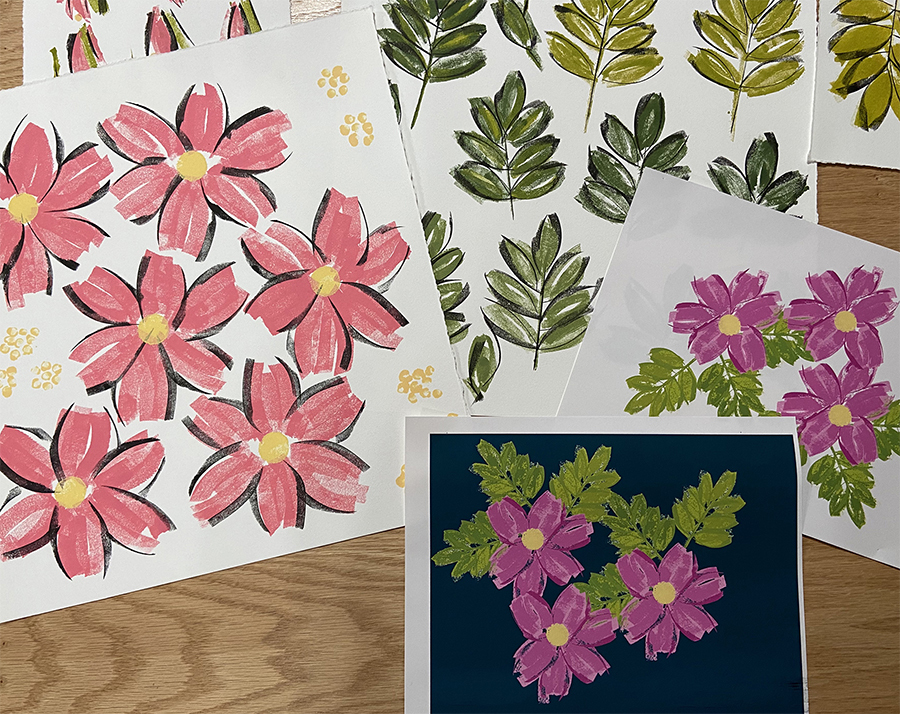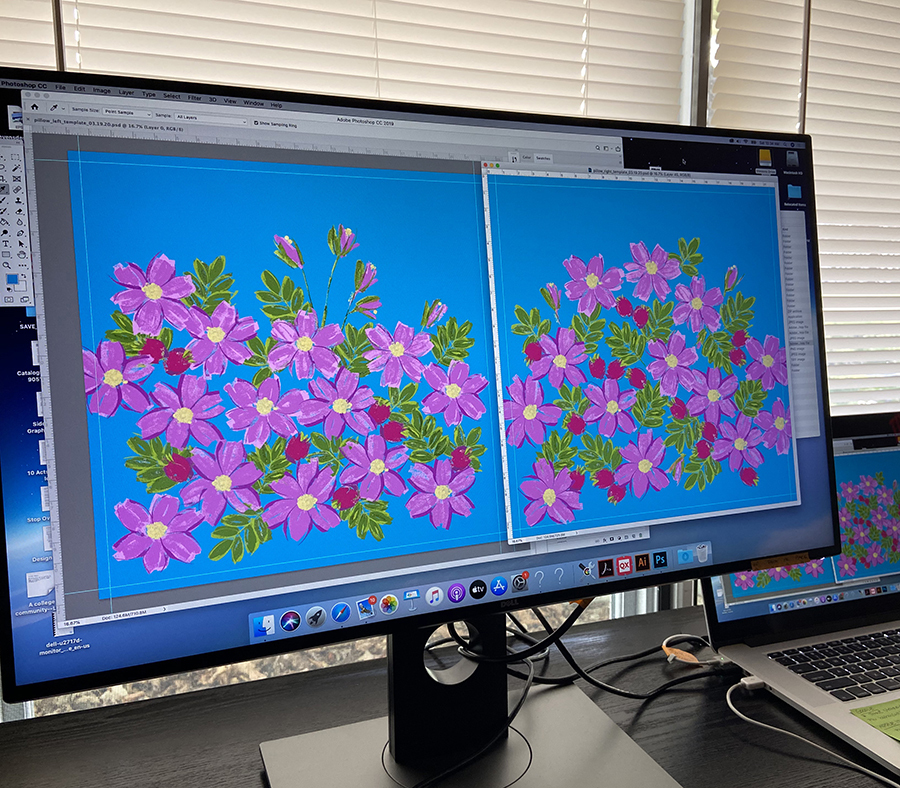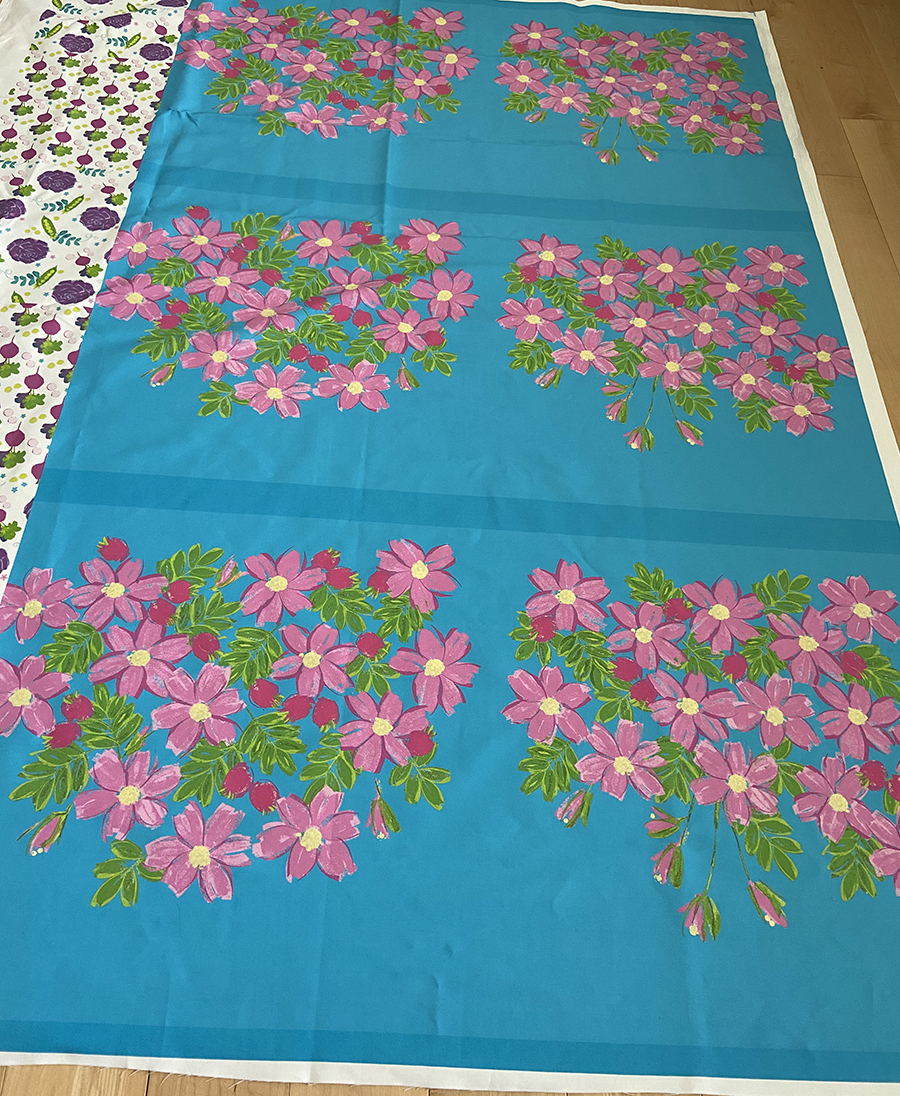table div table+table+table+table+table+table+table+table+table+table+table+table+table+table+table+table+table+table+table+table+table+table+table+table+table+table+table+table+table+table+table+table+table+table+table+table+table+table+table+table+table+table+table+table+table+table+table+table+table+table+table+table+table+table+table+table+table+table+table+table+table+table+table+table+table+table+table+table+table+table+table+table+table+table+table+table+table+table+table+table+table+table+table+table+table+table div table{width:100%;padding:0}table div table+table+table+table+table+table+table+table+table+table+table+table+table+table+table+table+table+table+table+table+table+table+table+table+table+table+table+table+table+table+table+table+table+table+table+table+table+table+table+table+table+table+table+table+table+table+table+table+table+table+table+table+table+table+table+table+table+table+table+table+table+table+table+table+table+table+table+table+table+table+table+table+table+table+table+table+table+table+table+table+table+table+table+table+table+table div table img{width:96.23%;padding:0;float:none}table div table+table+table+table+table+table+table+table+table+table+table+table+table+table+table+table+table+table+table+table+table+table+table+table+table+table+table+table+table+table+table+table+table+table+table+table+table+table+table+table+table+table+table+table+table+table+table+table+table+table+table+table+table+table+table+table+table+table+table+table+table+table+table+table+table+table+table+table+table+table+table+table+table+table+table+table+table+table+table+table+table+table+table+table+table+table div table td{width:100%;padding:0 1.88% 18px}/* styles *//* styles */ Vaune Trachtman "Lately, I’ve been working on pictures for my new series, “Now Is Always.” For this series, I’m combining my own images with pictures my father took in Philadelphia during the Depression. He was born in 1914 and died when I was five, so I only have a few memories of him. While working on this series I realized that he was alive during the Pandemic of 1918, and the series increasingly feels like a collaboration across time. I find myself growing closer to him even as with each passing day the time between us grows further apart. These are the thoughts that are informing “Now Is Always.” Here are two new pictures from the series. In other news, I had a two-week residency at The Vermont Studio Center in February. I have a couple of images in the SE Center for Photography’s alternative process show, juried by Jill Enfield. Also, my last series, “The Roaming Process,” is now available in the gallery store at The Print Center in Philadelphia.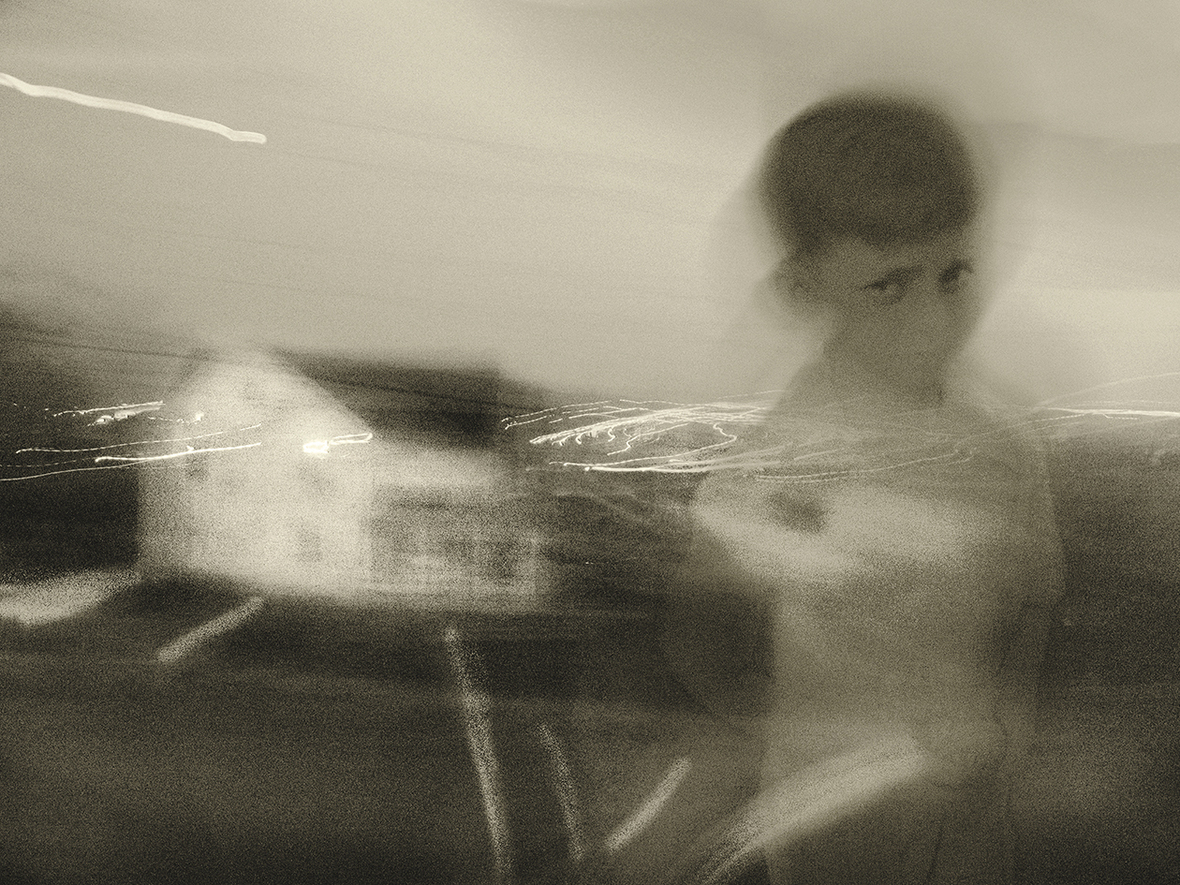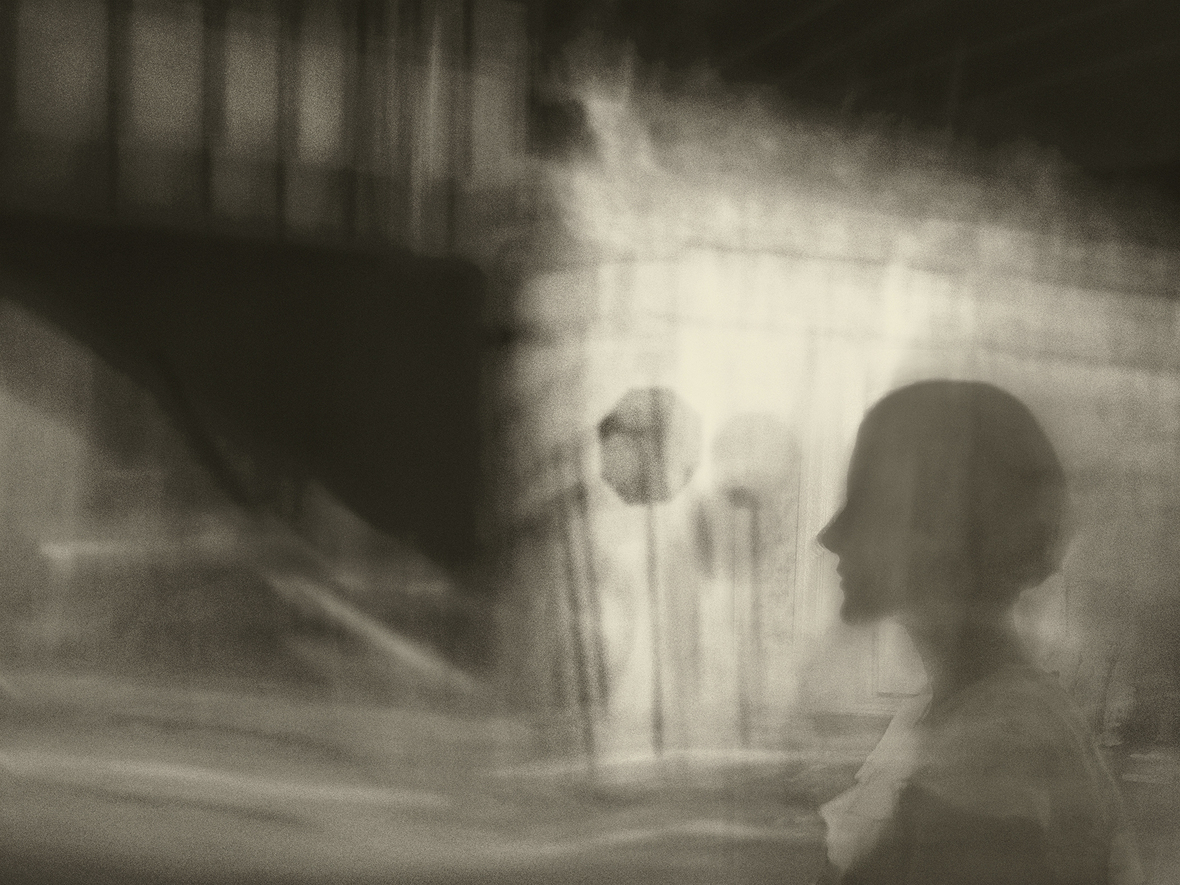table div table+table+table+table+table+table+table+table+table+table+table+table+table+table+table+table+table+table+table+table+table+table+table+table+table+table+table+table+table+table+table+table+table+table+table+table+table+table+table+table+table+table+table+table+table+table+table+table+table+table+table+table+table+table+table+table+table+table+table+table+table+table+table+table+table+table+table+table+table+table+table+table+table+table+table+table+table+table+table+table+table+table+table+table+table+table+table+table+table+table div table{width:100%;padding:0}table div table+table+table+table+table+table+table+table+table+table+table+table+table+table+table+table+table+table+table+table+table+table+table+table+table+table+table+table+table+table+table+table+table+table+table+table+table+table+table+table+table+table+table+table+table+table+table+table+table+table+table+table+table+table+table+table+table+table+table+table+table+table+table+table+table+table+table+table+table+table+table+table+table+table+table+table+table+table+table+table+table+table+table+table+table+table+table+table+table+table div table img{width:96.23%;padding:0;float:none}table div table+table+table+table+table+table+table+table+table+table+table+table+table+table+table+table+table+table+table+table+table+table+table+table+table+table+table+table+table+table+table+table+table+table+table+table+table+table+table+table+table+table+table+table+table+table+table+table+table+table+table+table+table+table+table+table+table+table+table+table+table+table+table+table+table+table+table+table+table+table+table+table+table+table+table+table+table+table+table+table+table+table+table+table+table+table+table+table+table+table div table td{width:100%;padding:0 1.88% 18px}/* styles *//* styles */ Lynn Peterfreund’s project, “Seeing Everyday” is happening online, although as our pandemic world settles, it may also happen in the A.P.E. gallery in the future. Exploring the idea of how we all see differently, especially people trained to see deeply in certain ways, “Seeing Everyday” is an offering of five or six weeks of online sessions. Starting April 27, Lynn is teaching a different drawing class every Monday at noon, and five or six guest presenters offer their perspectives on Wednesdays at noon beginning on Wed. April 29 with Joyce Silverstone. The full schedule will be posted on the A.P.E. Gallery website. Sessions are free, with a suggested donation to one of three charities (see A.P.E. website) Registration is required in order to received the zoom meeting info. Sign up: lynnpeterfreund@gmail.com This project is supported with a grant from the Mass. Cultural Council.
 table div table+table+table+table+table+table+table+table+table+table+table+table+table+table+table+table+table+table+table+table+table+table+table+table+table+table+table+table+table+table+table+table+table+table+table+table+table+table+table+table+table+table+table+table+table+table+table+table+table+table+table+table+table+table+table+table+table+table+table+table+table+table+table+table+table+table+table+table+table+table+table+table+table+table+table+table+table+table+table+table+table+table+table+table+table+table+table+table+table+table+table+table div table{width:100%;padding:0}table div table+table+table+table+table+table+table+table+table+table+table+table+table+table+table+table+table+table+table+table+table+table+table+table+table+table+table+table+table+table+table+table+table+table+table+table+table+table+table+table+table+table+table+table+table+table+table+table+table+table+table+table+table+table+table+table+table+table+table+table+table+table+table+table+table+table+table+table+table+table+table+table+table+table+table+table+table+table+table+table+table+table+table+table+table+table+table+table+table+table+table+table div table img{width:96.23%;padding:0;float:none}table div table+table+table+table+table+table+table+table+table+table+table+table+table+table+table+table+table+table+table+table+table+table+table+table+table+table+table+table+table+table+table+table+table+table+table+table+table+table+table+table+table+table+table+table+table+table+table+table+table+table+table+table+table+table+table+table+table+table+table+table+table+table+table+table+table+table+table+table+table+table+table+table+table+table+table+table+table+table+table+table+table+table+table+table+table+table+table+table+table+table+table+table div table td{width:100%;padding:0 1.88% 18px}/* styles */Like   Tweet   Pin   +1   in